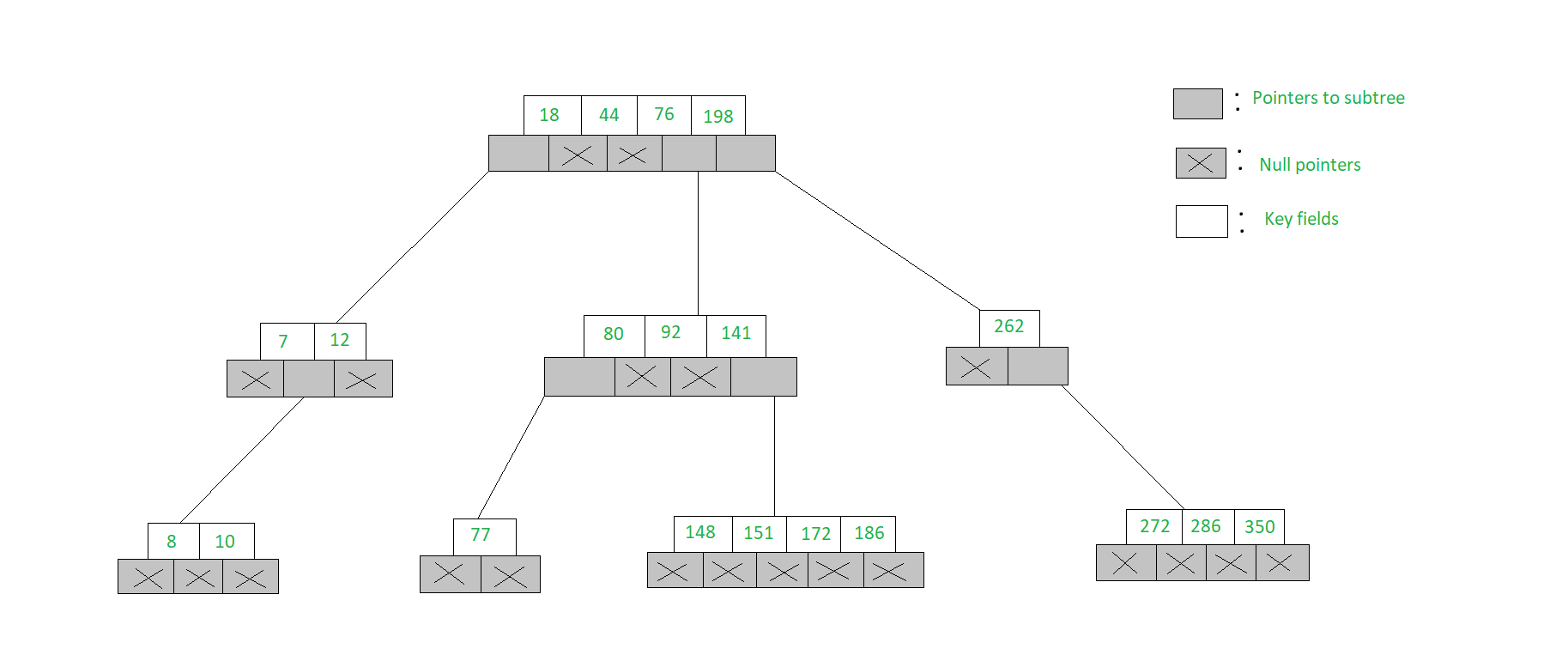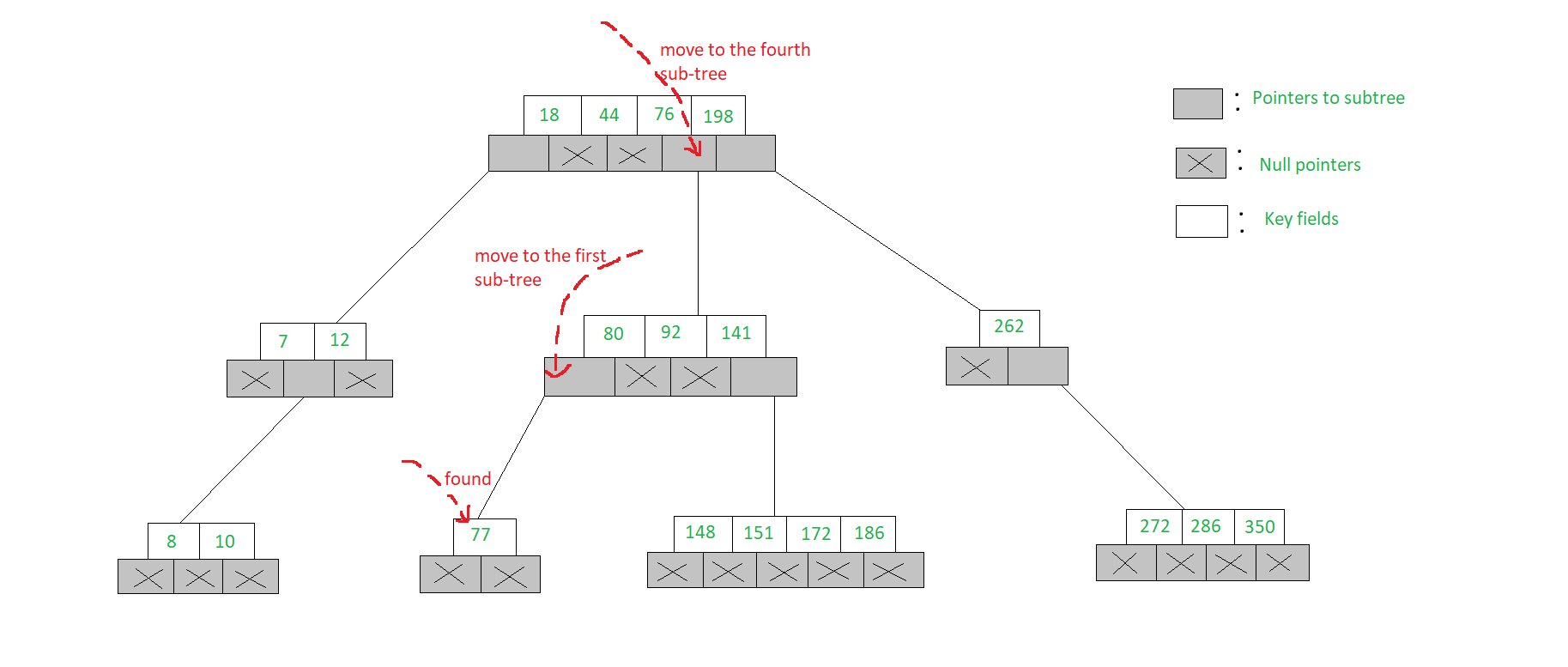Open in App
Not now

# m-WAY Search Trees | Set-1 ( Searching )

• Difficulty Level : Hard
• Last Updated : 10 Jan, 2023

The m-way search trees are multi-way trees which are generalised versions of binary trees where each node contains multiple elements. In an m-Way tree of order m, each node contains a maximum of m – 1 elements and m children.
The goal of m-Way search tree of height h calls for O(h) no. of accesses for an insert/delete/retrieval operation. Hence, it ensures that the height h is close to log_m(n + 1).
The number of elements in an m-Way search tree of height h ranges from a minimum of h to a maximum of.
An m-Way search tree of n elements ranges from a minimum height of log_m(n+1) to a maximum of n
An example of a 5-Way search tree is shown in the figure below. Observe how each node has at most 5 child nodes & therefore has at most 4 keys contained in it.The structure of a node of an m-Way tree is given below:

## C++

 `struct` `node {``    ``int` `count;``    ``int` `value[MAX + 1];``    ``struct` `node* child[MAX + 1];``};`

## Java

 `public` `class` `Node {``    ``int` `count;``    ``int``[] value = ``new` `int``[MAX + ``1``];``    ``Node[] child = ``new` `Node[MAX + ``1``];``}` `// This code is contributed by tapeshdua420.`

## Python3

 `class` `node:``    ``def` `__init__(``self``):``        ``self``.count ``=` `-``1``        ``self``.value ``=` `[``-``1``]``*``(``MAX` `+` `1``)``        ``self``.child ``=` `[``None``]``*``(``MAX` `+` `1``)`

## C#

 `class` `node {``    ``public` `int` `count;``    ``public` `int``[] value = ``new` `int``[MAX + 1];``    ``public` `node[] child = ``new` `node[MAX + 1];``}` `// This code is contributed by Tapesh (tapeshdua420)`

## Javascript

 `class Node {``  ``constructor() {``    ``this``.count = 0;``    ``this``.value = ``new` `Array(MAX + 1);``    ``this``.child = ``new` `Array(MAX + 1);``  ``}``}`

• Here, count represents the number of children that a particular node has
• The values of a node stored in the array value
• The addresses of child nodes are stored in the child array
• The MAX macro signifies the maximum number of values that a particular node can contain

Searching in an m-Way search tree:

• Searching for a key in an m-Way search tree is similar to that of binary search tree
• To search for 77 in the 5-Way search tree, shown in the figure, we begin at the root & as 77> 76> 44> 18, move to the fourth sub-tree
• In the root node of the fourth sub-tree, 77< 80 & therefore we move to the first sub-tree of the node. Since 77 is available in the only node of this sub-tree, we claim 77 was successfully searched## C++

 `// Searches value in the node``struct` `node* search(``int` `val,``                    ``struct` `node* root,``                    ``int``* pos)``{` `    ``// if root is Null then return``    ``if` `(root == NULL)``        ``return` `NULL;``    ``else` `{` `        ``// if node is found``        ``if` `(searchnode(val, root, pos))``            ``return` `root;` `        ``// if not then search in child nodes``        ``else``            ``return` `search(val,``                          ``root->child[*pos],``                          ``pos);``    ``}``}` `// Searches the node``int` `searchnode(``int` `val,``               ``struct` `node* n,``               ``int``* pos)``{``    ``// if val is less than node->value``    ``if` `(val < n->value) {``        ``*pos = 0;``        ``return` `0;``    ``}` `    ``// if the val is greater``    ``else` `{``        ``*pos = n->count;` `        ``// check in the child array``        ``// for correct position``        ``while` `((val < n->value[*pos])``               ``&& *pos > 1)``            ``(*pos)--;``        ``if` `(val == n->value[*pos])``            ``return` `1;``        ``else``            ``return` `0;``    ``}``}`

## Java

 `// Searches value in the node``public` `Node search(``int` `val, Node root, ``int` `pos) {``    ``// if root is Null then return``    ``if` `(root == ``null``)``        ``return` `null``;``    ``else` `{``        ``// if node is found``        ``if` `(searchnode(val, root, pos))``            ``return` `root;``            ` `        ``// if not then search in child nodes``        ``else``            ``return` `search(val, root.child[pos], pos);``    ``}``}` `// Searches the node``public` `boolean` `searchnode(``int` `val, Node n, ``int` `pos) {``    ``// if val is less than node.value``    ``if` `(val < n.value[``1``]) {``        ``pos = ``0``;``        ``return` `false``;``    ``}``     ``// if the val is greater``    ``else` `{``        ``pos = n.count;``        ` `        ``// check in the child array``        ``// for correct position``        ``while` `((val < n.value[pos]) && pos > ``1``)``            ``pos--;``            ` `        ``if` `(val == n.value[pos])``            ``return` `true``;``        ``else``            ``return` `false``;``    ``}``}` `// This code is contributed by Tapesh(tapeshdua420)`

## Python3

 `# Searches value in the node``def` `search(val, root, pos):` `    ``# if root is None then return``    ``if` `(root ``=``=` `None``):``        ``return` `None``    ``else` `:``        ``# if node is found``        ``if` `(searchnode(val, root, pos)):``            ``return` `root` `        ``# if not then search in child nodes``        ``else``:``            ``return` `search(val, root.child[pos], pos)``    `   `# Searches the node``def` `searchnode(val, n, pos):``    ``# if val is less than node.value``    ``if` `(val < n.value[``1``]):``        ``pos ``=` `0``        ``return` `0``    `  `    ``# if the val is greater``    ``else` `:``        ``pos ``=` `n.count` `        ``# check in the child array``        ``# for correct position``        ``while` `((val < n.value[pos]) ``and` `pos > ``1``):``            ``pos``-``=``1``        ``if` `(val ``=``=` `n.value[pos]):``            ``return` `1``        ``else``:``            ``return` `0``   `

## C#

 `// Searches value in the node``public` `Node search(``int` `val, Node root, ``int` `pos)``{``    ``// if root is Null then return``    ``if` `(root == ``null``)``        ``return` `null``;``    ``else` `{``        ``// if node is found``        ``if` `(searchnode(val, root, pos))``            ``return` `root;` `        ``// if not then search in child nodes``        ``else``            ``return` `search(val, root.child[pos], pos);``    ``}``}` `// Searches the node``public` `bool` `searchnode(``int` `val, Node n, ``int` `pos)``{``    ``// if val is less than node.value``    ``if` `(val < n.value) {``        ``pos = 0;``        ``return` `false``;``    ``}` `    ``// if the val is greater``    ``else` `{``        ``pos = n.count;` `        ``// check in the child array``        ``// for correct position``        ``while` `((val < n.value[pos]) && pos > 1)``            ``pos--;` `        ``if` `(val == n.value[pos])``            ``return` `true``;``        ``else``            ``return` `false``;``    ``}``}` `// This code is contributed by Tapesh (tapeshdua420)`

## Javascript

 `function` `search(val, root, pos) {``  ``// if root is null then return``  ``if` `(root === ``null``) {``    ``return` `null``;``  ``} ``else` `{``    ``// if node is found``    ``if` `(searchNode(val, root, pos)) {``      ``return` `root;``    ``}``    ``// if not then search in child nodes``    ``else` `{``      ``return` `search(val, root.child[pos.value], pos);``    ``}``  ``}``}` `function` `searchNode(val, n, pos) {``  ``// if val is less than node.value``  ``if` `(val < n.value) {``    ``pos.value = 0;``    ``return` `false``;``  ``}``  ``// if the val is greater``  ``else` `{``    ``pos.value = n.count;``    ``// check in the child array``    ``// for correct position``    ``while` `(val < n.value[pos.value] && pos.value > 1) {``      ``pos.value--;``    ``}``    ``if` `(val === n.value[pos.value]) {``      ``return` `true``;``    ``} ``else` `{``      ``return` `false``;``    ``}``  ``}``}`

search():

• The function search() receives three parameters
• The first parameter is the value to be searched, second is the address of the node from where the search is to be performed and third is the address of a variable that is used to store the position of the value once found
• Initially a condition is checked whether the address of the node being searched is NULL
• If it is, then simply a NULL value is returned
• Otherwise, a function searchnode() is called which actually searches the given value
• If the search is successful the address of the node in which the value is found is returned
• If the search is unsuccessful then a recursive call is made to the search() function for the child of the current node

searchnode():

• The function searchnode() receives three parameters
• The first parameter is the value that is to be searched
• The second parameter is the address of the node in which the search is to be performed and third is a pointer pos that holds the address of a variable in which the position of the value that once found is stored
• This function returns a value 0 if the search is unsuccessful and 1 if it is successful
• In this function initially it is checked whether the value that is to be searched is less than the very first value of the node
• If it is then it indicates that the value is not present in the current node. Hence, a value 0 is assigned in the variable that is pointed to by pos and 0 is returned, as the search is unsuccessful

My Personal Notes arrow_drop_up